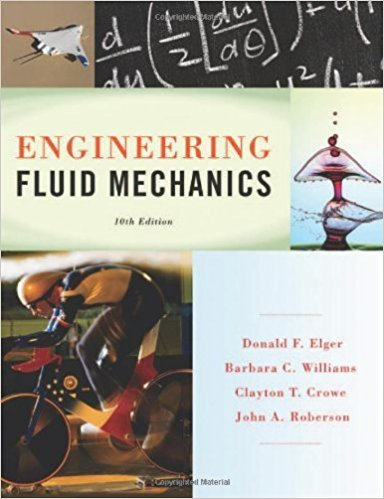×
×

# Calculate the pressure increase that must be applied to water to reduce its volume byISBN: 9781118164297 288

## Solution for problem 2.13 Chapter 2

Engineering Fluid Mechanics | 10th Edition

• Textbook Solutions
• 2901 Step-by-step solutions solved by professors and subject experts
• Get 24/7 help from StudySoup virtual teaching assistantsEngineering Fluid Mechanics | 10th Edition

4 5 1 349 Reviews
24
2
Problem 2.13

Calculate the pressure increase that must be applied to water to reduce its volume by 2%.

Step-by-Step Solution:
Step 1 of 3

Linear Momentum - Product if mass and velocity of an object - ⃗ = ⃗ - SI unit: kg*m/s - A vector - The total momentum of a system of objects is the vector sum of the momenta of all the objects - ⃗⃗⃗⃗⃗⃗⃗⃗⃗⃗⃗⃗⃗⃗ +⃗⃗⃗⃗⃗⃗⃗⃗⃗⃗ + ⋯ 1 2 3 Momentum and Newton’s Second Law ⃗ - Ʃ = ⃗ - The net...

Step 2 of 3

Step 3 of 3

##### ISBN: 9781118164297

Unlock Textbook Solution